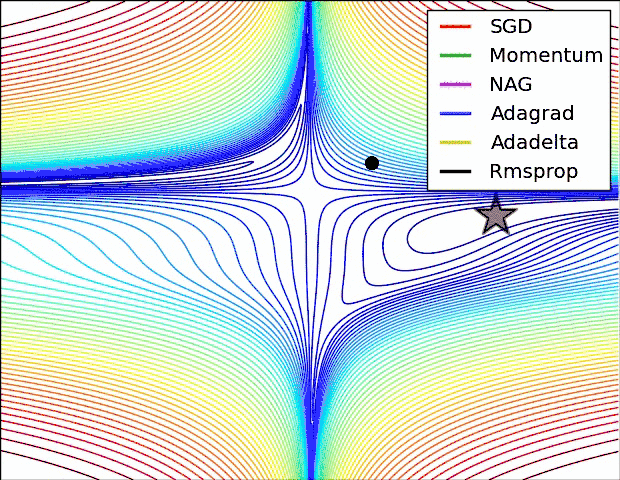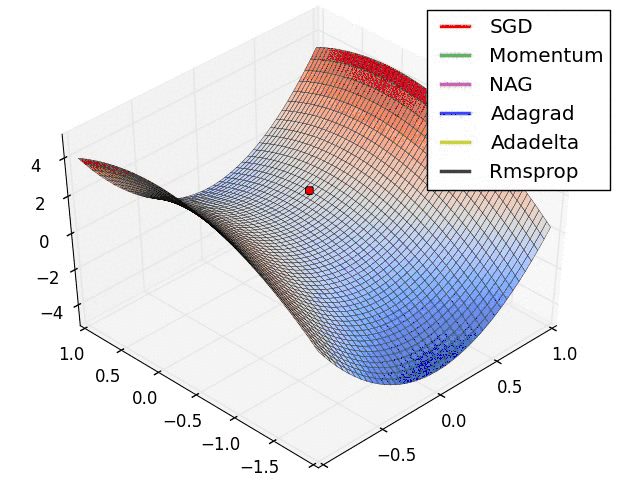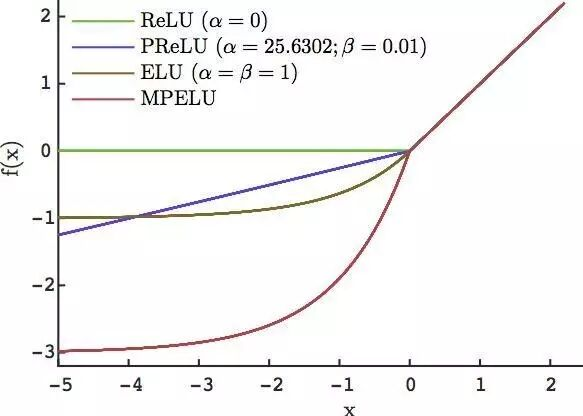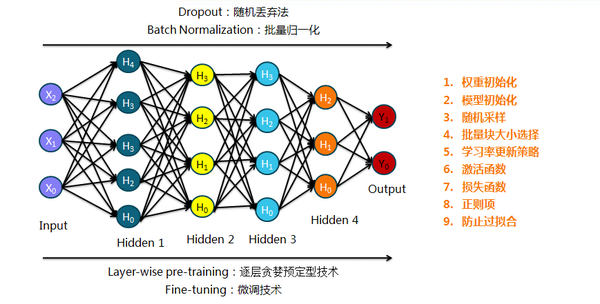## Building Blocks

finding the optimum in parameter space

Strategies:

• Batch: $\theta = \theta - \eta \cdot \nabla_ \theta J(\theta)$
• Stochastic: $\theta = \theta - \eta \cdot \nabla_\theta J(\theta;x_i;y_i)$, faster but biased
• Mini-batch: $\theta = \theta - \eta \cdot \nabla_\theta J(\theta;x_{i:i+m};y_{i:i+m})$, intermediate

Challenges:

• $\eta$: apply learning rate schedules
• Saddle points as local minimum: spiking NN for quantum tunneling?

Improvements for SDG:

• add momentum: $v_t = \gamma v_t - 1 + \eta \cdot \nabla_\theta J(\theta); \theta = \theta -v_t$, reduce damping
• NAG, Nesterov accelerated gradient: add correction based on momentum, $J(\theta) -> J(\theta - \gamma v_t - 1)$, avoid momentum crash
• Adagrad, adaptive gradient: $\eta_i$ for each $\theta_i$, $\theta_{t+1}=\theta_t - \frac{\eta}{\sqrt{G_t+\epsilon}}\odot g_t$
• Adadelta: reduce the calculation of $G_t$ with a window-based average, $E_t$
• RMSprop: $E_t = \gamma E_{t-1} + (1-\gamma)g_t^2$
• Adam, adaptive moment estimation: bias-corrected from weighted average mean and varianceAsynchronous SGD for parallelization:

• Hogwild
• Downpour SGD
• Delay-tolerant Algorithms for SGD
• TensorFlow
• EASGD, elastic Averaging SGD: local variable fluctuate from center variable

Extra to explore:

• Shuffling and Curriculum Learning
• Batch normalization: keep N(0,1)
• Early stopping: avoid overfitting
• Gradient noise: shuffle away from local

### Activation Function

back-propagatable, non-linear projection

keep nonlinearity & non-vanishing gradient

• Sigmoid: $f(x) = \frac{1}{1 + e^{-x}}$, work with cross-entropy cost function $y\ln{a}+(1-y)\ln{(1-a)}$ to avoid gradient vanishing
• tanh, $tanh(x)= 2 \sigmoid(2x)-1$: better but not the final solution
• ReLU, $y=x\ge0? x:0$
• constant gradient on one side
• output shift & hard to converge
• PReLU, $f(y_i)= y_i>0? y_i: a_iy_i$: regulate the left side
• Maxout, $\max{w_ix+b_i}$
• ELU, $f(x)= x>0?x:\alpha (\exp(x)-1)$
• Noisy Activation Functions, Gulcehre, C., et al., Noisy Activation Functions, in ICML 2016. 2016
• CReLU, pair-grouping phenomenon
• MPELUPractical tips:

### Regularization

minimize the loss function with reasonable complexity

Supervised learning: $w^* = \arg\min_w \sum_i L(y_i, f(x_i;w)) + \lambda \Omega(w)$, solve ill-conditioned matrix

L0/L1, minimize the absolute sum: Lasso regularization, make $w$ sparse, $||w||_1 \le C$, linear bound

• Feature Selection: less feature needed
• Interpretability: less complex

less features

L2, minimize the square sum: Ridge Regression/Weight Decay, $||w||_2 \le C$, quadratic bound

won't reduce features
but reduce certain feature dependence

### Tuning Methods

the evil approach## Neural Networks• Feedforward Neural Network: word2vec
• Huffman tree
• CBOW: words -> word
• skip-gram: word -> words
• Denoising Autoencoders: obtain good representation
• perform noise mapping: $x \rightarrow \tilde{ x}$
• keep loss as $\mathcal{L}(x,\tilde{x'})$
• Restricted Boltzmann Machine: unsupervised learning
• generative approach: obtain $P(X,Y)$ for P(Y|X); in contrast of discriminative approach, which only cares about $P(Y|X)$
• methods: Markov process & Gibbs sampling
• metrics: KL divergence, Shannon entropy, $\sum_i P(i)\log\frac{P(i)}{Q(i)}$
• probability distribution: $P = \frac{1}{Z}e^{-E(v,h)}$
• energy function: $E(v,h) =-v^T Wh -a^Tv -b^Th$, $v$ visible unit, $h$ hidden unit
• Generative Adversarial Network: Turing learning
• discriminator: convolutional
• generator: deconvolutional
• applications
• Residual Neural Network
• plain layer + shortcuts
• residual function: $\mathcal{F}(x):=h(x) - x$
• shortcut: $y=w_s x + \mathcal{F}(x, {w_i})$
• Convolutional Neural Network: computer vision
• convolutional layer with kernels
• Recurrent Neural Network
• LSTM: a memory cell $c_t$, an input gate $i_t$, an 'output' gate $o_t$ and a forget gate $f_t$. $x_t \rightarrow h_t$• Attention: potential of memory
• applications### Spiking Neural Network (3rd generation)

Spiking neuron: accumulate their activation into a potential over time, and only send out a signal (a "spike") when this potential crosses a threshold and the neuron is reset.

Integrator: memory or nonlinear response

### Extras

Published: Thu 05 July 2018. By Dongming Jin in